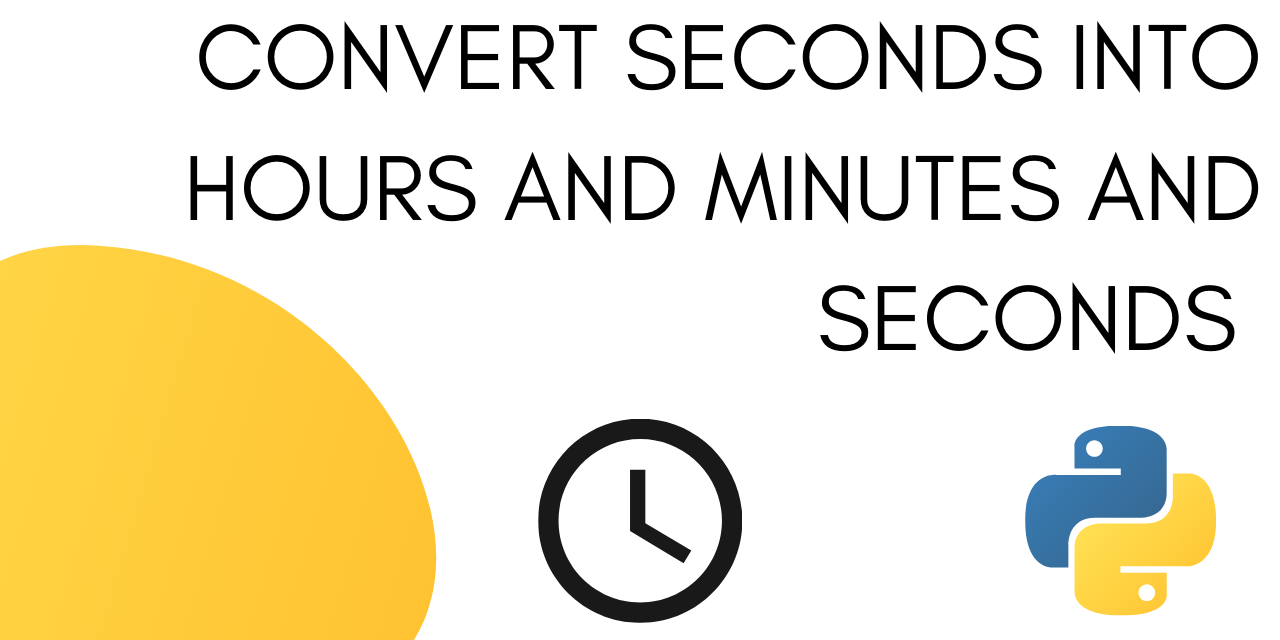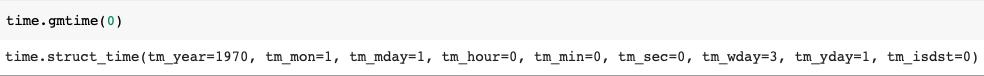# Convert time into hours minutes and seconds in Python

Published on August 3, 2022By Jayant VermaWhile we believe that this content benefits our community, we have not yet thoroughly reviewed it. If you have any suggestions for improvements, please let us know by clicking the “report an issue“ button at the bottom of the tutorial.

In this tutorial, we will be talking about time. Don’t worry, this isn’t a boring history tutorial, rather we will be looking at different ways of converting time in seconds to time in hours, minutes, and seconds.

Moving forwards we will be referring to time in hours, minutes and seconds as time in the preferred format.

It will look like :

``````2:46:40
``````

Let’s take some ‘time’ and think about the problem at hand. No doubt python has amazing modules to do the conversion for us. But let’s try and write our own program first before we move on to the in-built modules.

## Building a Custom function to convert time into hours minutes and seconds

To write our own conversion function we first need to think about the problem mathematically.

How do you convert seconds into the preferred format?

You need to get the value of hours, minutes and seconds.

We are going to assume that the time in seconds doesn’t exceed the total number of seconds in a day. If it does we will divide it with the total number of seconds in a day and take the remainder.

This is mathematically represented as :

``````seconds = seconds % (24 * 3600)
``````

% operator gives the remainder.

24*3600 since one hour has 3600 seconds (60*60) and one day has 24 hours.

After this we can proceed and calculate the hour value from seconds.

### 1. Get the hour value

To get the hour value from seconds we will be using the floor division operator (//).

It returns the integral part of the quotient.

Since we need the number of hours, we will divide the total number of seconds (n) by the total number of seconds in an hour (3600).

Mathematically this is represented as :

``````hour = seconds // 3600
``````

After this we need to calculate the minutes.

### 2. Get the minute value

To calculate the value of minutes we need to first divide the total number of seconds by 3600 and take the remainder.

Mathematically this is represented as :

`````` seconds = seconds % 3600
``````

Now to calculate the value of minutes from the above result we will again use the floor operator.

``````minutes = seconds // 60
``````

A minute has sixty seconds hence we floor the seconds value with 60.

After calculating minute value we can move forward to calculate the seconds’ value for our preferred format.

### 3. Get the seconds value

To get seconds value we again need to divide the total number of seconds by the number of seconds in one minute (60) and take the remainder.

Mathematically this is done as follows :

`````` seconds = seconds % 60
``````

This will give the second value that we need for our preferred format.

### 4. Complete code

Let’s compile the above knowledge in a python function.

``````def convert_to_preferred_format(sec):
sec = sec % (24 * 3600)
hour = sec // 3600
sec %= 3600
min = sec // 60
sec %= 60
print("seconds value in hours:",hour)
print("seconds value in minutes:",min)
return "%02d:%02d:%02d" % (hour, min, sec)

n = 10000
print("Time in preferred format :-",convert(n))
``````

Output :

``````seconds value in hours: 2
seconds value in minutes: 46
Time in preferred format :- 02:46:40
``````

## Using the Time module

Now let’s look at an inbuilt module that lets us convert seconds into our preferred format in one line of code.

The time module defines the epoch as January 1, 1970, 00:00:00 (UTC) in Unix systems (system dependent). Epoch is basically the start of time for a computer. Think of it as day 0. Whenever we convert seconds using the time module, this epoch is used as the reference point.

To output the epoch in your system, use the following line of code :

``````time.gmtime(0)
``````To convert seconds into preferred format use the following line of code:

``````time.strftime("%H:%M:%S", time.gmtime(n))
``````

This line takes the time in seconds as ‘n’ and then lets you output hour, minute, and second value separately.

The complete python code is as follows:

``````import time
n=10000
time_format = time.strftime("%H:%M:%S", time.gmtime(n))
print("Time in preferred format :-",time_format)

``````

Output :

``````Time in preferred format :- 02:46:40
``````

The time module also gives you the option to display some extra information such as day, month, and year.

`%a` display abbreviated weekday name.
`%A` display full weekday name.
`%b` display abbreviated month name.
`%B` display full month name.
`%c` display the appropriate date and time representation.
`%d` display day of the month as a decimal number [01,31].

Let’s try using %a and %b.

``````import time
n=100000000000
time_format = time.strftime("Day: %a, Time: %H:%M:%S, Month: %b", time.gmtime(n))
print("Time in preferred format :-",time_format)
``````

Output :

``````Time in preferred format :- Day: Wed, Time: 09:46:40, Month: Nov
``````

## Using Datetime module

You can also use the timedelta method under the DateTime module to convert seconds into the preferred format.

It displays the time as days, hours, minutes, and seconds elapsed since the epoch.

The python code to convert seconds into the preferred format using Datetime module is as follows:

``````import datetime
n= 10000000
time_format = str(datetime.timedelta(seconds = n))
print("Time in preferred format :-",time_format)
``````

Output :

``````Time in preferred format :- 115 days, 17:46:40
``````

## Conclusion

This tutorial looked at three different ways you can use to convert seconds into hours, minutes, and seconds. Broadly there are two different ways to go about the problem.

Either you write your own function or use an inbuilt module. We started by writing our own function and then looked at the time and DateTime module.

Thanks for learning with the DigitalOcean Community. Check out our offerings for compute, storage, networking, and managed databases.Jayant Verma

author

#### Still looking for an answer?

Ask a questionSearch for more help

Click below to sign up and get \$200 of credit to try our products over 60 days!

### Popular Topics

##### Join the Tech Talk##### Hollie's Hub for Good

Working on improving health and education, reducing inequality, and spurring economic growth? We’d like to help.